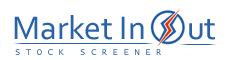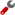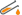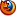Please enable JavaScript to view this page content properly Log In | Sign Up
 Fundamental Analysis
FUNDAMENTAL ANALYSIS

Overview

Fundamental analysis is the study of economic, industry, and company conditions in an effort to determine the value of a company's stock. Fundamental analysis typically focuses on key statistics in a company's financial statements to determine if the stock price is correctly valued.

I realize that some people will find a discussion on fundamental analysis within a book on technical analysis peculiar, but the two theories are not as different as many people believe. It is quite popular to apply technical analysis to charts of fundamental data, for example, to compare trends in interest rates with changes in security prices. It is also popular to use fundamental analysis to select securities and then use technical analysis to time individual trades. Even diehard technicians can benefit from an understanding of fundamental analysis (and vice versa).

Interpretation

Most fundamental information focuses on economic, industry, and company statistics. The typical approach to analyzing a company involves four basic steps:

1. Determine the condition of the general economy.

2. Determine the condition of the industry.

3. Determine the condition of the company.

4. Determine the value of the company's stock.

Economic Analysis

The economy is studied to determine if overall conditions are good for the stock market. Is inflation a concern? Are interest rates likely to rise or fall? Are consumers spending? Is the trade balance favorable? Is the money supply expanding or contracting? These are just some of the questions that the fundamental analyst would ask to determine if economic conditions are right for the stock market.

Industry Analysis

The company's industry obviously influences the outlook for the company. Even the best stocks can post mediocre returns if they are in an industry that is struggling. It is often said that a weak stock in a strong industry is preferable to a strong stock in a weak industry.

Company Analysis

After determining the economic and industry conditions, the company itself is analyzed to determine its financial health. This is usually done by studying the company's financial statements. From these statements a number of useful ratios can be calculated. The ratios fall under five main categories: profitability, price, liquidity, leverage, and efficiency. When performing ratio analysis on a company, the ratios should be compared to other companies within the same or similar industry to get a feel for what is considered "normal." At least one popular ratio from each category is shown below.

Net Profit Margin.A company's net profit margin is a profitability ratio calculated by dividing net income by total sales. This ratio indicates how much profit the company is able to squeeze out of each dollar of sales. For example, a net profit margin of 30%, indicates that \$0.30 of every \$1.00 in sales is realized in profits.

P/E Ratio. The P/E ratio (i.e., Price/Earnings ratio) is a price ratio calculated by dividing the security's current stock price by the previous four quarter's earnings per share (EPS).

The P/E Ratio shows how much an investor must pay to "buy" \$1 of the company's earnings. For example, if a stock's current price is \$20 and the EPS for the last four quarters was \$2, the P/E ratio is 10 (i.e., \$20 / \$2 = 10). This means that you must pay \$10 to "buy" \$1 of the company's earnings. Of course, investor expectations of company's future performance play a heavy role in determining a company's current P/E ratio.

A common approach is to compare the P/E ratio of companies within the same industry. All else being equal, the company with the lower P/E ratio is the better value.

Book Value Per Share. A company's book value is a price ratio calculated by dividing total net assets (assets minus liabilities) by total shares outstanding. Depending on the accounting methods used and the age of the assets, book value can be helpful in determining if a security is overpriced or under-priced. If a security is selling at a price far below book value, it may be an indication that the security is under-priced.

Current Ratio. A company's current ratio is a liquidity ratio calculated by dividing current assets by current liabilities. This measures the company's ability to meet current debt obligations. The higher the ratio the more liquid the company. For example, a current ratio of 3.0 means that the company's current assets, if liquidated, would be sufficient to pay for three times the company's current liabilities.

Debt Ratio. A company's debt ratio is a leverage ratio calculated by dividing total liabilities by total assets. This ratio measures the extent to which total assets have been financed with debt. For example, a debt ratio of 40% indicates that 40% of the company's assets have been financed with borrowed funds. Debt is a two-edged sword. During times of economic stress or rising interest rates, companies with a high debt ratio can experience financial problems. However, during good times, debt can enhance profitability by financing growth at a lower cost.

Inventory Turnover. A company's inventory turnover is an efficiency ratio calculated by dividing cost of goods sold by inventories. It reflects how effectively the company manages its inventories by showing the number of times per year inventories are turned over (replaced). Of course, this type of ratio is highly dependent on the industry. A grocery store chain will have a much higher turnover than a commercial airplane manufacturer. As stated previously, it is important to compare ratios with other companies in the same industry.

Stock Price Valuation

After determining the condition and outlook of the economy, the industry, and the company, the fundamental analyst is prepared to determine if the company's stock is overvalued, undervalued, or correctly valued.

Several valuation models have been developed to help determine the value of a stock. These include dividend models which focus on the present value of expected dividends, earnings models which focuses on the present value of expected earnings, and asset models which focus on the value of the company's assets.

There is no doubt that fundamental factors play a major role in a stock's price. However, if you form your price expectations based on fundamental factors, it is important that you study the price history as well or you may end up owning an undervalued stock that remains undervalued.

 P / E Stock Screener Action Price / Earnings Ratio: More than 50Price / Earnings Ratio: 25 to 50Price / Earnings Ratio: 15 to 25Price / Earnings Ratio: 10 to 15Price / Earnings Ratio: 5 to 10Price / Earnings Ratio: 0 to 5Best viewed in